Graph IDEData Graphics ► Trajectory

A trajectory is a sequence of points connected by curves. The curves are Cubic Bezier sections (see Cubic Bezier). The figures below show examples of trajectories.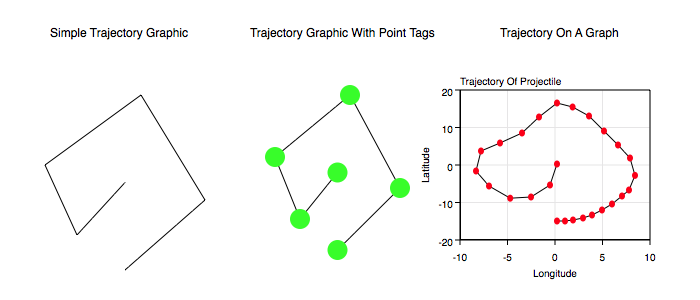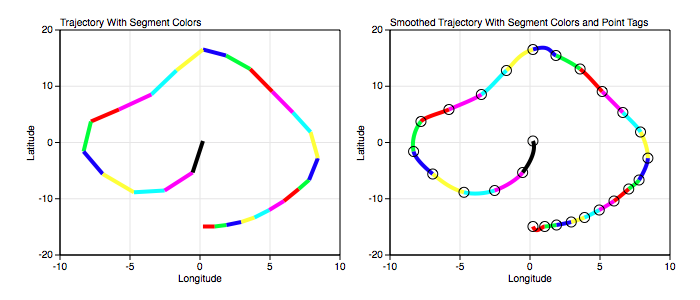Some standard operations are itemized below.

• To create a trajectory bring forward the Graphic Selector, click the trajectory factory cell and then mouse down on a Graphic View and drag the cursor to another point. The initial and final cursor locations define the boundary of a predefined trajectory graphic.

• You can also create trajectories from the standard Palettes menu item or drag them out from the trajectory Factory Inspector.

• Resizing and rotating the trajectory transforms all points simultaneously. For more information see Standard Editing.

• One thing you will probably want to do with a trajectory is modify its points. You can do that via the main or parser Inspector Editor or by editing it directly with the mouse controls. Initially, the spline knots (tangent end points) are under the vertex points so you will not be able to get at them by clicking on them. You should first smooth out the graphic so that the control knots move away from the vertex points and then enter the mouse edit mode and move those knots as you wish.

• To program a trajectory see the Programming section.

Data Editor

The Data Editor for the Trajectory Graphic is shown below.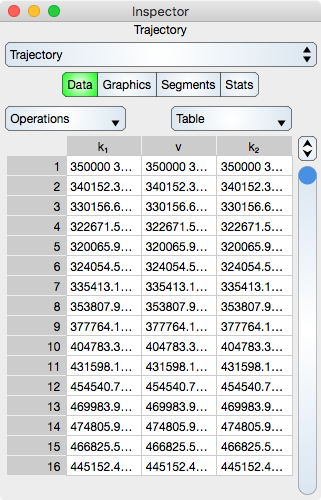Trajectory Specific Controls

Operations : Select this to perform common sequence operations upon the data. The most important sequence operation is Switch X and Y because that transposes the data and hence the dimensions. Contrast this to the Function graphic which assumes that x is alway ascending and always the independent variable of the coordinate system (graph).

Table

Table controls are described in the Tables section.

The rows represent vertex and knot values. While in Atomic mode, the cell represents a point (x and y value) and while in component mode the cell represents either a x or y value. Hence, in atomic mode there are three column while in component mode there are six columns.

Graphics Editor

The Graphics Editor for the Trajectory graphic is shown below.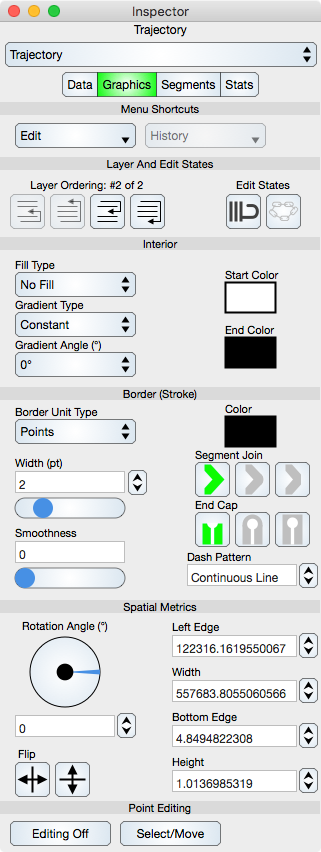Common Controls

Controls common to all graphics are described in the Graphics section.

Trajectory Specific Controls

Smoothness : Adjust the spline knots of the Trajectory so that the tangent lines at a vertex are more coincidental. Zero moves the knot locations under the vertex location while one is maximum smoothness. Choosing a smoothness greater than zero is a good way to expose the knots for point editing.

Point Editing

Editing Off/On : Places the graphic into or out of edit mode. While in edit mode the vertices are shown by indicators and can be adjusted using mouse or touch events. Double-clicking the graphic also toggles this edit mode.

Select/Move or Add/Delete : Select/Move mode permits the vertex editing to select and move those locations while Add/Delete mode will delete a vertex if they are hit or add a vertex if a Trajectory segment is hit. This can also be accomplished using the shift key if available.

Segments Editor

The Segments Editor for the Trajectory graphic is shown below.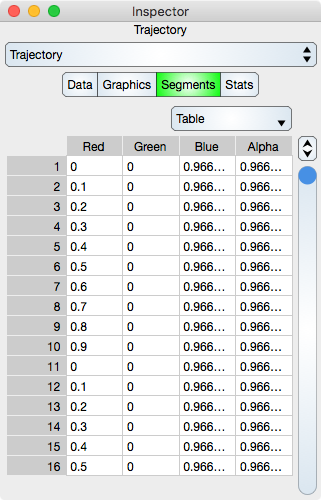Segments are the parametric Cubic Bezier segments between adjacent vertices. See Sequence Colors for additional information.

Table

Table controls are described in the Tables section.

The rows represent a segment color values. While in Atomic mode, the cell represents a rgba value and while in component mode the cell represents either single r, g, b and a. Hence, in atomic mode there is one column while in component mode there are four columns.

Note that if the Data sequence is resorted then the Segments sequence will not be resorted.

Stats Editor

The Stats Editor for the Trajectory graphic is shown below. Note: The data is consider the vertex points while the knots are considered as graphical. Thus the statistics only reflect the vertex values.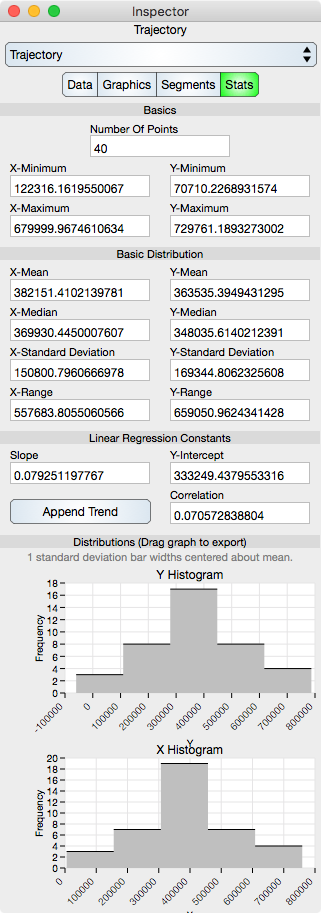Basics

Number Of Points : Shows the number of points in the Trajectory.

X-Minimum : x-minimum value of all the data points.

Y-Minimum : y-minimum value of all the data points.

X-Maximum : x-maximum value of all the data points.

Y-Maximum : y-maximum value of all the data points.

Basic Distribution

The basic distribution is shown separately for the x-dimension and the y-dimension.

Mean : Shows the mean of the data.

Median : Shows the median of the data.

Standard Deviation : Shows the standard deviation of the data.

Range : Shows the range of the data (maximum - minimum).

Linear Regression Constants

Note that linear regression may also be referred to as line fit.

Slope : Shows the slope of the linear regression.

Y-Intercept : Shows the y-intercept of the linear regression.

Correlation : Shows the correlation constant of the linear regression.

Distributions

Y Histogram : Shows the y-histogram of the data. This graph can be dragged onto the Graphic View.

X Histogram : Shows the x-histogram of the data. This graph can be dragged onto the Graphic View.